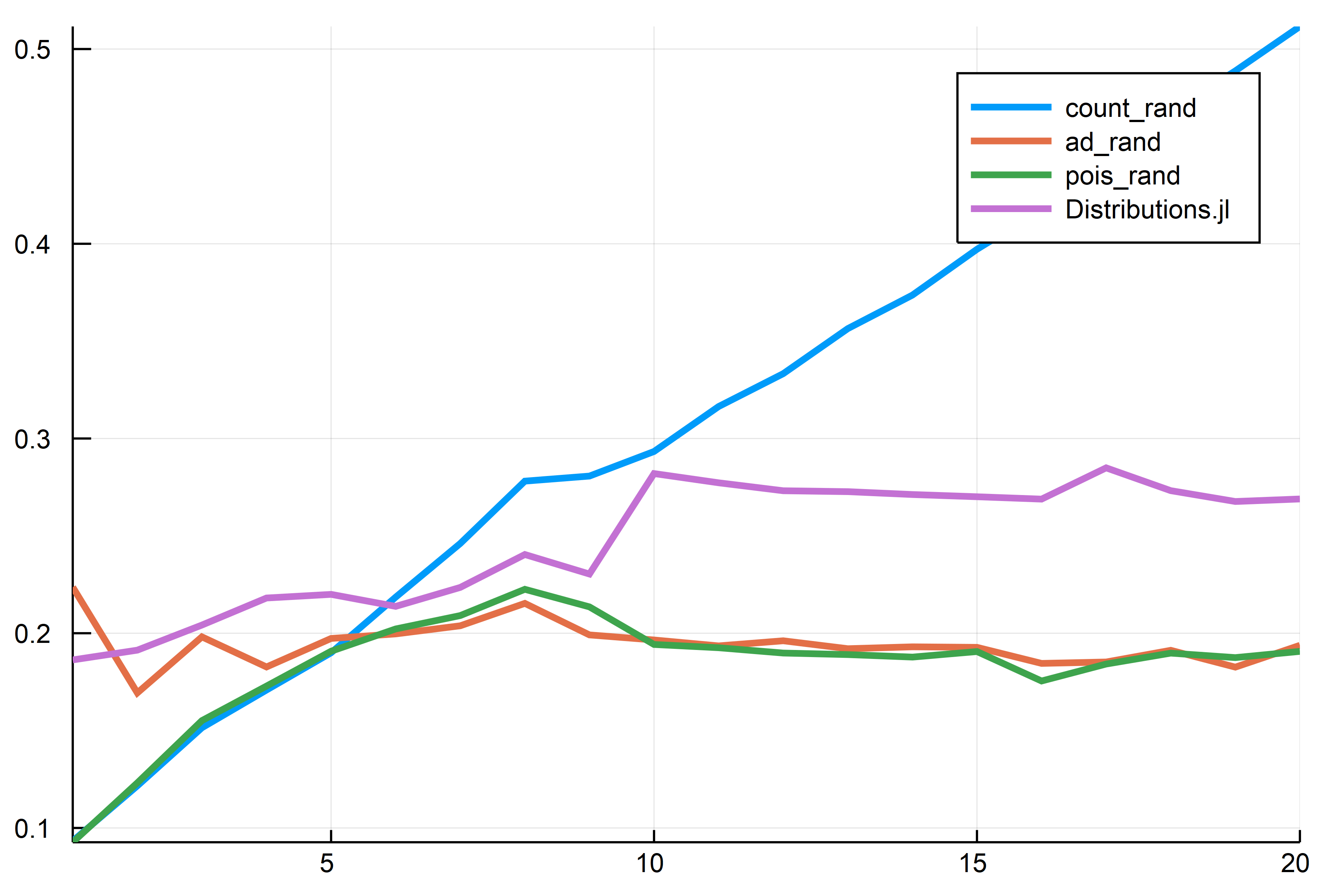{{ message }}
/ PoissonRandom.jl Public

Fast Poisson Random Numbers in pure Julia for scientific machine learning (SciML)

# SciML/PoissonRandom.jl

Switch branches/tags
Nothing to show

## Files

Failed to load latest commit information.
Type
Name
Commit time

# PoissonRandom.jl: Fast Poisson Random Numbers## Tutorials and Documentation

For information on using the package, see the stable documentation. Use the in-development documentation for the version of the documentation, which contains the unreleased features.

## Usage

```Pkg.add("PoissonRandom")

# Simple Poisson random
pois_rand(λ)

# Using another RNG
using RandomNumbers
rng = Xorshifts.Xoroshiro128Plus()
pois_rand(rng,λ)```

## Implementation

It mixes two methods. A simple count method and a method from a normal approximation. See this blog post for details.

## Benchmark

```using RandomNumbers, Distributions, BenchmarkTools, StaticArrays,
RecursiveArrayTools, Plots, PoissonRandom
rng = Xorshifts.Xoroshiro128Plus()

function n_count(rng,λ,n)
tmp = 0
for i in 1:n
tmp += PoissonRandom.count_rand(rng,λ)
end
end

function n_pois(rng,λ,n)
tmp = 0
for i in 1:n
tmp += pois_rand(rng,λ)
end
end

tmp = 0
for i in 1:n
end
end

function n_dist(λ,n)
tmp = 0
for i in 1:n
tmp += rand(Poisson(λ))
end
end

function time_λ(rng,λ,n)
t1 = @elapsed n_count(rng,λ,n)
t3 = @elapsed n_pois(rng,λ,n)
t4 = @elapsed n_dist(λ,n)
@SArray [t1,t2,t3,t4]
end

# Compile
time_λ(rng,5,5000000)
# Run with a bunch of λ
times = VectorOfArray([time_λ(rng,n,5000000) for n in 1:20])'
plot(times,labels = labels, lw = 3)```So this package ends up about 30% or so faster than Distributions.jl (the method at the far edge is λ-independent so that goes on forever).

Fast Poisson Random Numbers in pure Julia for scientific machine learning (SciML)

## Releases 6

Change argument order Latest
Jan 20, 2019